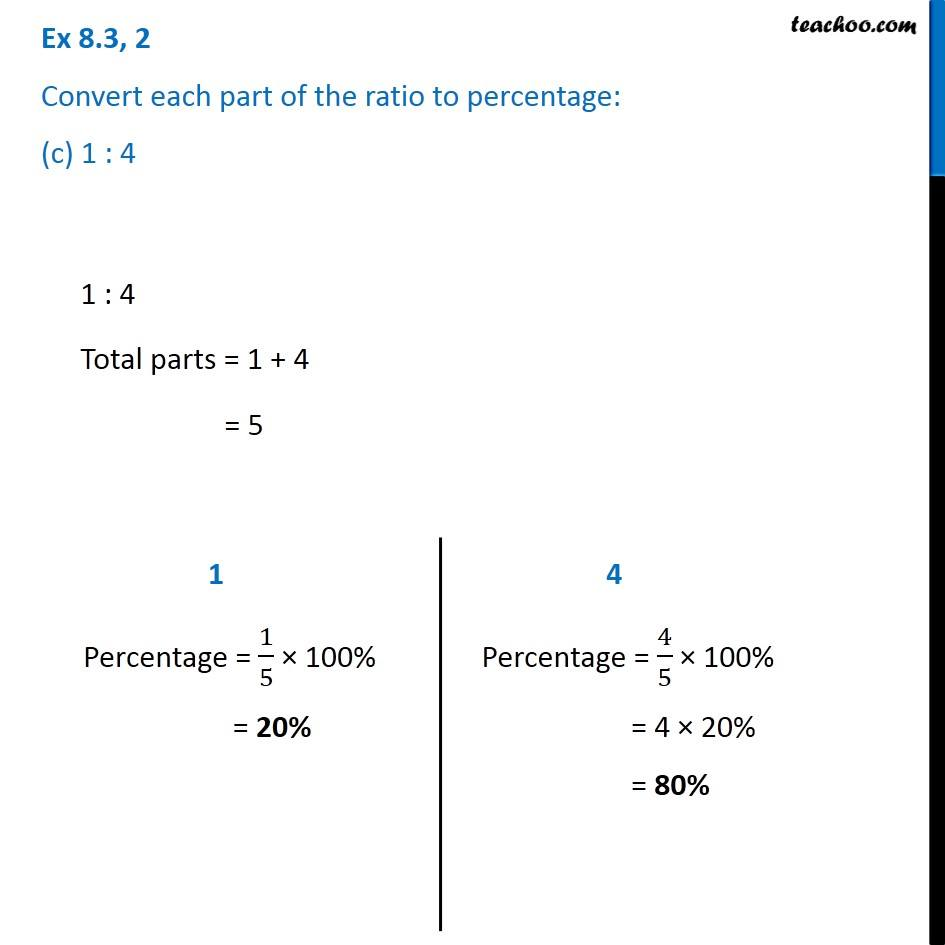Ex 8.3

Chapter 8 Class 7 Comparing Quantities
Serial order wiseGet live Maths 1-on-1 Classs - Class 6 to 12

### Transcript

Ex 8.3, 2 Convert each part of the ratio to percentage: (c) 1 : 4 1 : 4 Total parts = 1 + 4 = 5 1 Percentage = 1/5 × 100% = 20% 4 Percentage = 4/5 × 100% = 4 × 20% = 80%